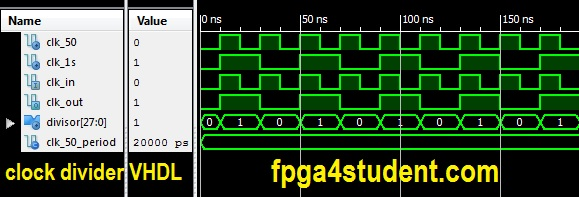# VHDL Code for Clock Divider on FPGA

## This VHDL project presents a full VHDL code for clock divider on FPGA. Testbench VHDL code for clock divider is also provided. The VHDL code for the clock divider is synthesizable and verified on FPGA.### VHDL code for Clock divider on FPGA:

```-- fpga4student.com FPGA projects, VHDL projects, Verilog projects
-- VHDL project: VHDL code for digital clock on FPGA
library IEEE;
use IEEE.STD_LOGIC_1164.ALL;
USE IEEE.STD_LOGIC_UNSIGNED.ALL;
entity clock_div is
port (
clk_in: in std_logic; -- clock input on FPGA
clk_out: out std_logic -- clock output
);
end clock_div;

architecture Behavioral of clock_div is
signal divisor: std_logic_vector(27 downto 0):=(others =>'0');
begin
process(clk_in)
begin
if(rising_edge(clk_in)) then
divisor <= divisor + x"0000001";
-- If(divisor>=x"2FAF07F") then -- for running on FPGA -- comment when running simulation
-- Modify the divisor (x"2FAF07F"=49999999) above to get the clock frequency you want:
-- Frequency of clk_out = Frequency of (clk_in) divided by (divisor + 1)
-- If the frequency of clk_in is 50MHz and the divisor is 49999999=x"2FAF07F",
-- the frequency of clk_out is 1Hz```
``` if(divisor>=x"0000001") then -- for running simulation -- comment when running on FPGA
-- divisor = 1 => divide clock by half for simulation purposes
divisor <= x"0000000";
end if;```
``` if(divisor<x"0000001") then -- replace x"0000001" by x"17D7840" when running on FPGA   clk_out <= '1'; else  clk_out <= '0'; end if; end if;
end process;```
```end Behavioral;

```

### VHDL Testbench code for clock divider on FPGA:

```-- fpga4student.com FPGA projects, VHDL projects, Verilog projects
-- VHDL project: VHDL code for clock divider on FPGA
-- VHDL Testbench code for clock divider
LIBRARY ieee;
USE ieee.std_logic_1164.ALL;

ENTITY tb_clock_div IS
END tb_clock_div;

ARCHITECTURE behavior OF tb_clock_div IS ```
```    -- Clock divider
COMPONENT clock_div
PORT(
clk_in : IN  std_logic;
clk_out : OUT  std_logic
);
END COMPONENT;
--Inputs
signal clk_50 : std_logic := '0';

--Outputs
signal clk_1s : std_logic;

-- Clock period definitions
constant clk_50_period : time := 20 ns;

BEGIN

-- Instantiate the VHDL clock divider
uut: clock_div PORT MAP (
clk_in => clk_50,
clk_out => clk_1s
);

-- creating clock
clk_50_process :process
begin
clk_50 <= '0';
wait for clk_50_period/2;
clk_50 <= '1';
wait for clk_50_period/2;
end process;

-- Stimulus process
stim_proc: process
begin
wait for 100 ns;
wait for clk_50_period*10;
wait;
end process;

END;```

#### Simulation Waveform for clock divider on FPGA:#### In the VHDL code for simulation purposes, the divisor is set to be 1 so the clock frequency of clk_out is obtained by dividing the frequency of clk_in by 2 as explained in the main VHDL code of the clock divider. The simulation waveform also shows the frequency of clk_in is half of the clock frequency of clk_in. When you run the VHDL code on FPGA, you can modify the clock divisor in the code to get the frequency that you want from the clock input on FPGA.

It is noted that this code is about to create another clock in your design, so the FPGA tools required to take care of an extra internally generated clock during clock tree synthesis, which might cause FPGA timing issues as it is not generated by dedicated FPGA clock generators (PLL/DCM/etc). In addition, you also have to take care of the multi-clock domain issues while designing such as interfacing signals between different clock domains (synchronizer needed, etc). It is recommended on FPGA to generate a slower clock enable signal instead to drive another part of your design. You can visit here for more details on how to do it in VHDL.
Verilog code for Clock divider on FPGA: here